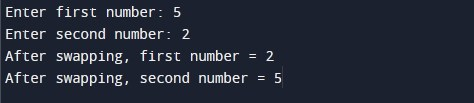C Program to Swap two numbers without third variable

In this tutorial you will learn about the C Program to Swap two numbers without third variable and its application with practical example.

In this tutorial, we will learn to create a program to swap two numbers without using temporary variable in C programming language.

Prerequisites

Before starting with this tutorial we assume that you are best aware of the following C programming topics:

• C Data Types
• C Variables
• C Input Output
• C Operators

Program to Swap Numbers Without Using Temporary Variables

In this program we will swap two integer numbers without using temporary variable. We would first declared and initialized the required variables. Next, we would prompt user to input two integer numbers. Later in the program we will swap numbers without using a temporary variable. Finally, we will display number values after swapping of numbers.

Output:-In the above program, we have first declared and initialized a set variables required in the program.

• num1 = it holds the first integer number value
• num2 = it holds the second integer number value

In the next statement user will be prompted to enter the two integer number values which will be assigned to variable ‘num1’ and ‘num2’ respectively. Next, we will swap two integer numbers without using a temporary variable. Finally, we will display number values after swapping of numbers.

In this tutorial we have learn about the C Program to Swap two numbers without third variable and its application with practical example. I hope you will like this tutorial.